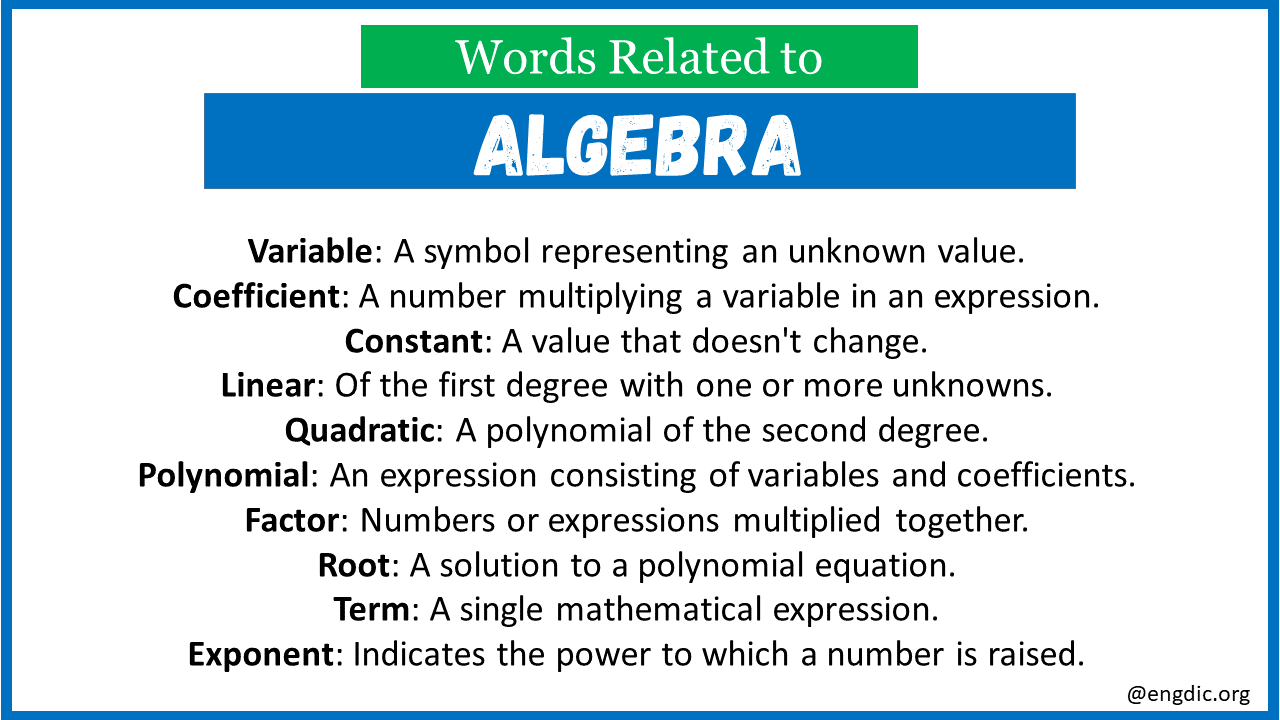# Top 30 Words Related to Algebra

Algebra, a branch of mathematics, involves symbols and rules for manipulating those symbols. It’s about finding the unknown or representing quantities in abstract forms. To better understand algebra, it’s essential to familiarize oneself with its most pertinent terminology. Here are the top 30 words related to algebra.

## Words Related to Algebra

Here are the top 30 terms related to algebra with meanings:

1. Variable: A symbol representing an unknown value.
2. Equation: A mathematical statement asserting two expressions are equal.
3. Coefficient: A number multiplying a variable in an expression.
4. Constant: A value that doesn’t change.
5. Linear: Of the first degree with one or more unknowns.
6. Quadratic: A polynomial of the second degree.
7. Polynomial: An expression consisting of variables and coefficients.
8. Factor: Numbers or expressions multiplied together.
9. Root: A solution to a polynomial equation.
10. Term: A single mathematical expression.
11. Exponent: Indicates the power to which a number is raised.
12. Binomial: A polynomial with two terms.
13. Monomial: A polynomial with one term.
14. Trinomial: A polynomial with three terms.
15. Matrix: A rectangular array of numbers or symbols.
16. Determinant: A value computed from a square matrix.
17. Inverse: Opposite in effect or nature.
18. Function: A relation between input and output values.
19. Domain: The set of possible input values.
20. Range: The set of possible output values.
21. Rational Expression: A fraction with polynomials in the numerator and denominator.
22. Absolute Value: The distance a number is from zero.
23. Radical: Represents the root of a number.
24. Factorization: Expressing a number as a product of its factors.
25. Algebraic Expression: Combination of variables, numbers, and operations.
26. Inequality: A relation stating one value is less than or greater than another.
27. Solve: Finding the value or values that make an equation true.
28. Simplification: Reducing an expression to its simplest form.
29. Substitution: Replacing a variable with a value or expression.
30. Base: The number in exponentiation being raised to a power.

Explore More Related Words:

Words Related to Alcohol

Words Related to Air Travel

Words Related to Air Transportation